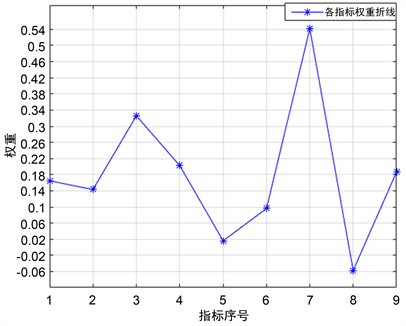# 本科教育质量的综合评估——以江苏省为例Comprehensive Evaluation of the Quality of Undergraduate Education—Take Jiangsu Province for Example

DOI: 10.12677/ASS.2020.97141, PDF, HTML, XML, 下载: 29  浏览: 83

Abstract: Based on Matlab software, this paper builds a system of comprehensive evaluation model, including the comprehensive evaluation model based on improved principal component analysis, based on correlation analysis and the weight of index model and the indicators based on virtual control city delete and key indicator selection model, and application of the system to solve a series of undergraduate education quality evaluation problems. Combined with the modeling results, this paper points out the current situation of undergraduate education quality in Jiangsu Province, and puts forward three constructive policy suggestions to improve the quality of undergraduate education in Jiangsu Province.

1. 引言

2. 基于改进的主成分分析方法的综合评价模型

2.1. 指标选取及量化处理

Table 1. Index quantization processing table

2.2. 数据初步分析Table 2. Sum, mean and variance of data of each index number

Table 3. The correlation coefficient matrix of the original data of each index

1) 对原始数据进行标准化处理，将各指标值aij转化为标准化指标值 ${a}_{ij}^{*}$ ，得到矩阵A*

${a}_{ij}^{*}=\frac{{a}_{ij}-{\stackrel{¯}{a}}_{j}}{{s}_{j}},\text{\hspace{0.17em}}\text{\hspace{0.17em}}i=1,2,\cdots ,n;\text{\hspace{0.17em}}j=1,2,\cdots ,m$

2) 计算A*的样本协方差矩阵S。

3) 求出S的特征值为 ${\lambda }_{\text{1}}\ge {\lambda }_{\text{2}}\ge \cdots \ge {\lambda }_{m}\ge 0$ ，相应于 ${\lambda }_{i}$ 的正交化单位特征向量为 ${v}_{i}=\left[{v}_{i}{}_{\text{1}},{v}_{i}{}_{\text{2}},\cdots ,{v}_{im}\right]$ ，则A*的第i个主成分为 ${y}_{i}={v}_{i}x$ ，其中x为m维观测向量，即 $x={\left[{x}_{\text{1}},{x}_{\text{2}},\cdots ,{x}_{m}\right]}^{\text{T}}$$i=1,2,\cdots ,m$

4) 选择主成分，计算综合评价值。

a) 计算特征值 ${\lambda }_{i}\left(i=\text{1},\text{2},\cdots ,m\right)$ 的信息贡献率bi。其中

${b}_{i}=\frac{{\lambda }_{i}}{\underset{j=1}{\overset{m}{\sum }}{\lambda }_{i}},\text{\hspace{0.17em}}\text{\hspace{0.17em}}i=\text{1},2,\cdots ,m$ (2.1)

b) 累积贡献率βk足够大(一般取βk = 0.85, 0.90, 0.95)时，则选择前k个主成分。其中

${\beta }_{k}=\frac{\underset{i=1}{\overset{k}{\sum }}{\lambda }_{i}}{\underset{i=1}{\overset{m}{\sum }}{\lambda }_{i}},\text{\hspace{0.17em}}\text{\hspace{0.17em}}k=\text{1},2,\cdots ,m$ (2.2)

c) 最后计算出综合得分，并据此进行测评

$G=\underset{i=1}{\overset{k}{\sum }}{b}_{i}{y}_{i}$ (2.3)

2.3. 基于改进的主成分分析方法的评价模型的建立

2.3.1. 原始数据的预处理方法的改进1——原始数据的均值化

${c}_{ij}=\frac{{a}_{ij}}{{\stackrel{¯}{a}}_{j}},\text{\hspace{0.17em}}\text{\hspace{0.17em}}i=1,2,\cdots ,n;\text{\hspace{0.17em}}j=1,2,\cdots ,m$ (2.4)

2.3.2. 原始数据的预处理方法的改进2——数据的一致化处理

${c}_{ij}^{*}=\mathrm{max}\left({c}_{j}\right)-{c}_{ij}$ (2.5)

${c}_{ij}^{*}={c}_{ij}$

2.3.3. 综合评价方法的改进——熵值法

a) 计算第j个主成分下第i个样本的比重： ${p}_{ij}=\frac{{d}_{ij}}{\underset{i=1}{\overset{n}{\sum }}{d}_{ij}}$(2.6)

b) 计算第j个主成分的熵值： ${e}_{j}=-\frac{1}{\mathrm{ln}n}\underset{i=1}{\overset{n}{\sum }}{p}_{ij}\mathrm{ln}{p}_{ij}$(2.7)

c) 计算第j个主成分的权重： ${b}_{j}^{*}=\frac{1-{e}_{j}}{\underset{j=1}{\overset{r}{\sum }}\left(1-{e}_{j}\right)}$(2.8)

d) 计算样本的综合值： $G=\underset{j=1}{\overset{r}{\sum }}{b}_{j}^{*}{y}_{j}$ ，最后按综合值对样本进行排序即可。

2.4. 模型应用——江苏省地级市的本科教育质量的评估与排名Table 4. Improved principal component analysis resultsTable 5. The eigenvectors corresponding to the first four principal components of the meanized variable

Table 6. Principal component score matrix

$G=\underset{i=1}{\overset{r}{\sum }}{b}_{i}^{*}{y}_{i}=0.4097{y}_{1}+0.5567{y}_{2}-0.1528{y}_{3}+0.1863{y}_{4}$ (2.9)Table 7. The comprehensive evaluation value and ranking result of undergraduate education quality in each cityFigure 1. The comprehensive evaluation value and ranking result

3. 基于相关性分析和权重的指标删除模型

3.1. 指标的初步删除

3.1.1. 指标间的相关性

${r}_{p,q}=\frac{n\underset{i=1}{\overset{n}{\sum }}{c}_{ip}^{*}{c}_{iq}^{*}-\underset{i=1}{\overset{n}{\sum }}{c}_{ip}^{*}\underset{i=1}{\overset{n}{\sum }}{c}_{iq}^{*}}{\sqrt{n{\underset{i=1}{\overset{n}{\sum }}{c}_{ip}^{*2}-\left(\underset{i=1}{\overset{n}{\sum }}{c}_{ip}^{*}\right)}^{2}}\sqrt{n{\underset{i=1}{\overset{n}{\sum }}{c}_{iq}^{*2}-\left(\underset{i=1}{\overset{n}{\sum }}{c}_{iq}^{*}\right)}^{2}}}$ (3.1)

3.1.2. 指标的权重

$G=\underset{i=1}{\overset{r}{\sum }}{b}_{i}^{*}{y}_{i}\text{}=\underset{i}{\overset{r}{\sum }}{b}_{i}^{*}{u}_{i}x$ (3.2)Table 9. The weight of each index to the comprehensive evaluation of urban undergraduate education qualityFigure 2. The weight of each index to the comprehensive evaluation of urban undergraduate education quality

3.1.3. 指标的初步删除Table 10. The ranking after deleting the double top disciplineTable 11. The ranking after deleting teaching conditions and utilizationTable 12. The ranking after deleting the construction and teaching condition and utilization of double top disciplines

3.2. 指标删除与关键指标提取模型的建立

3.2.1. 虚拟控制城市的引入

${c}_{0j}^{*}=\frac{1}{n}\underset{i=1}{\overset{n}{\sum }}{c}_{ij}^{*}$ (3.3)

3.2.2. 指标灵敏度的定量计算

1) 综合得分的均值化处理

${{G}^{\prime }}_{ij}=\frac{{G}_{ij}}{{\stackrel{¯}{G}}_{j}},\text{\hspace{0.17em}}\text{\hspace{0.17em}}i=1,2,\cdots ,n;\text{\hspace{0.17em}}j=1,2,\cdots ,m$ (3.4)

2) 计算灵敏度

${D}_{j}=\underset{i=1}{\overset{n}{\sum }}{\left({{G}^{\prime }}_{ij}-{G}_{i,0}\right)}^{2}$ (3.5)

3.3. 指标删除与关键指标提取模型的求解Table 13. The index data of virtual cityTable 14. The comprehensive evaluation value and ranking result of undergraduate education quality in each city after inserting virtual cityTable 15. The overall score of each city after changing the number of universities in the virtual controlled cityTable 16. Nine indicators of sensitivity

4. 对江苏省本科教育的政策与建议

4.1. 江苏省的本科教育现状

4.2. 政策建议

a) 在保持南京市本科教育优势的同时，鼓励南京市高校对省内其余各市的援助与指导，提高各市高校的科研投入与产出比，这样可以起到均衡教育资源的作用。

b) 江苏省有关部分应该加大对南京市以外各市高校的科研、校园建设等资金的投入，使其得以在科研经费、人才引进、学生培养等方面有更多的资本。

c) 江苏省各高校要重视双一流学科的建设，抓住双一流学科建设对本科教育质量影响较小的机会，努力建设好双一流学科。双一流学科是国家重点关注对象，所以日后的影响必然会增大。因此未来本科教育质量的评估也会有所改变。

  教育部办公厅文件(教高厅2号). 教育部办公厅关于开展普通高等学校本科教学工作合格评估的通知[EB/OL]. http://www.moe.gov.cn/srcsite/A08/s7056/201802/t20180208_327138.html, 2011-05-14.  百度百科. 本科院校[EB/OL]. https://baike.baidu.com/item/%E6%9C%AC%E7%A7%91%E9%99%A2%E6%A0%A1/17606093?fr=aladdin, 2020-04-02.  百度百科. 专任老师[EB/OL]. https://baike.baidu.com/item/%E4%B8%93%E4%BB%BB%E6%95%99%E5%B8%88/4799208?fr=aladdin, 2020-04-01.  高等教育质量监测国家数据平台. 江苏省2019年质量报告[EB/OL]. http://udb.heec.edu.cn/passport/portal/index.html?returnUrl=http%3A%2F%2Fudb.heec.edu.cn%2Fweb%2F%3Fv%3D1525135884729, 2020-04-29.  曲双红, 李华, 李刚. 基于主成分分析的几种常用改进方法[J]. 统计与决策, 2017(5): 155-156.  叶双峰. 关于主成分分析做综合评价的改进[J]. 数理统计与管理, 2011(2): 10-17.  王学民. 对主成分分析综合得分方法的质疑[J]. 统计与决策, 2007(4): 56-61.